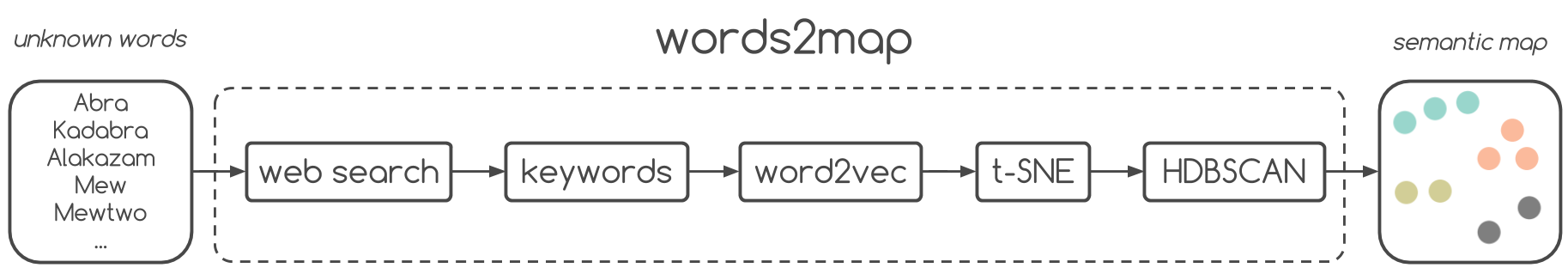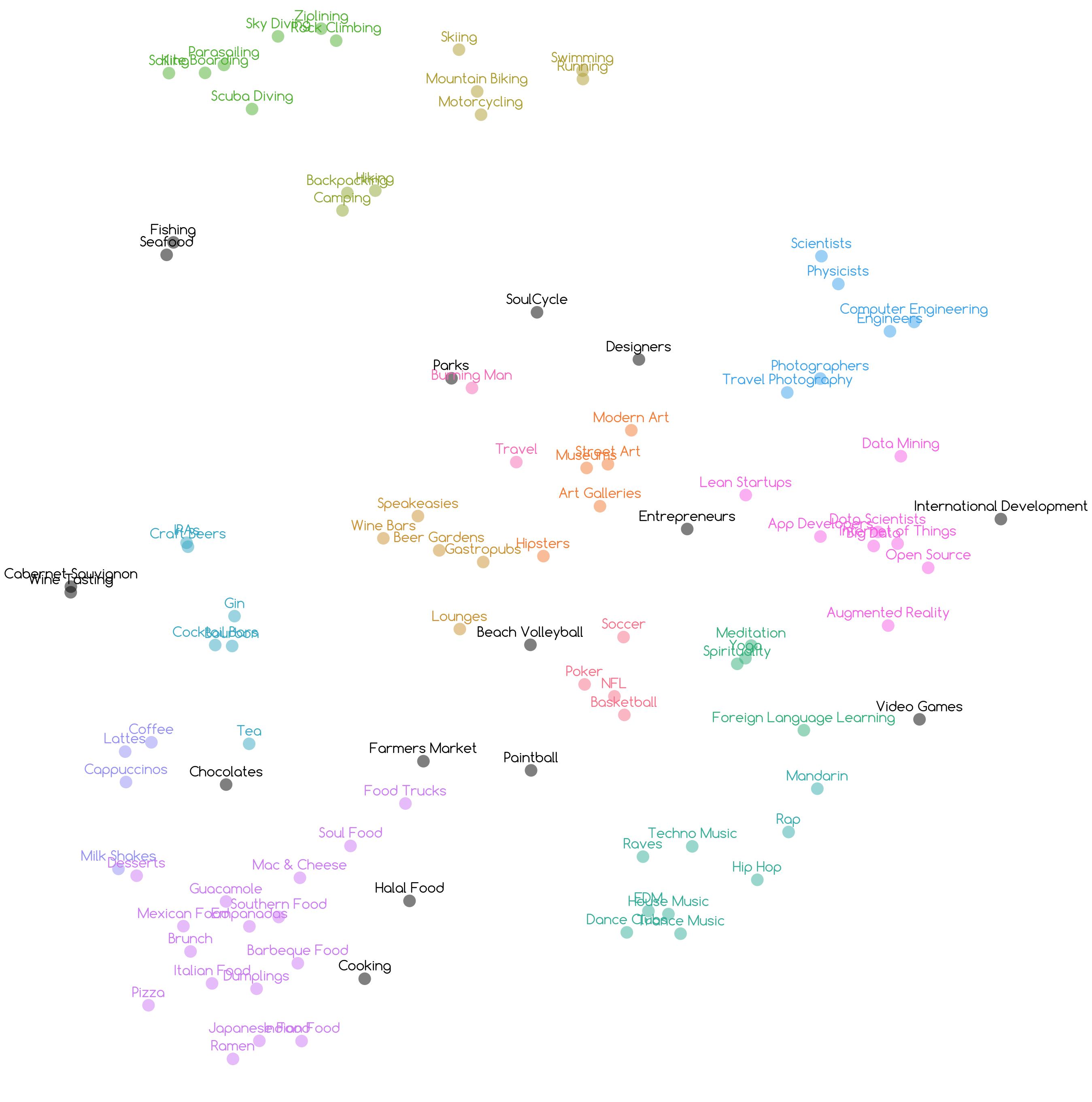### Install

``````git clone https://github.com/overlap-ai/words2map.git
cd words2map
./install.sh``````

### Derive new vectors for words by searching online

``````from words2map import *
model = load_model()
words = load_words("passions.csv")
vectors = [derive_vector(word, model) for word in words]
save_derived_vectors(words, vectors, "passions.txt")``````

### Analyze derived word vectors

``````from words2map import *
from pprint import pprint
model = load_derived_vectors("passions.txt")
pprint(k_nearest_neighbors(model=model, k=10, word="Data_Scientists"))``````

### Visualize clusters of vectors

``````from words2map import *
model = load_derived_vectors("passions.txt")
words = [word for word in model.vocab]
vectors = [model[word] for word in words]
vectors_in_2D = reduce_dimensionality(vectors)
generate_clusters(words, vectors_in_2D)``````